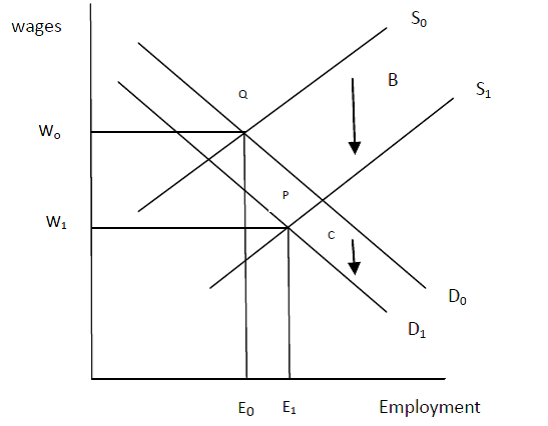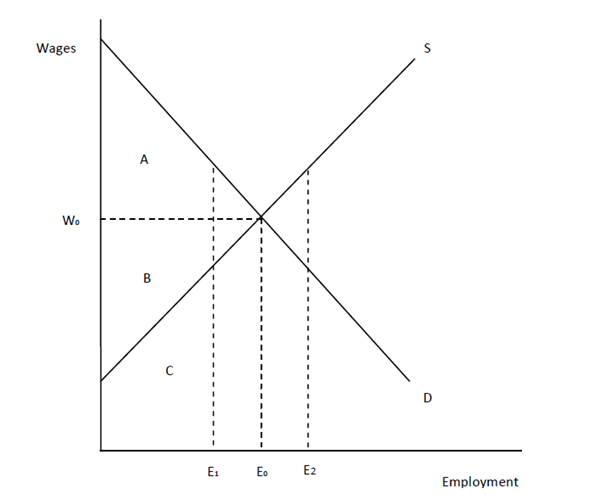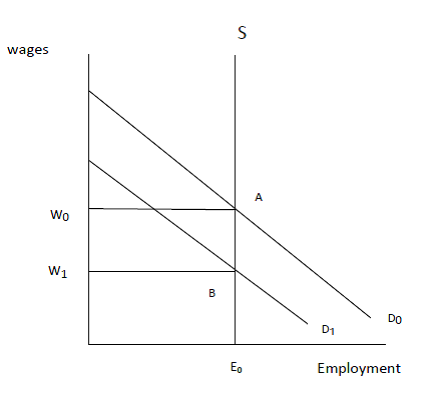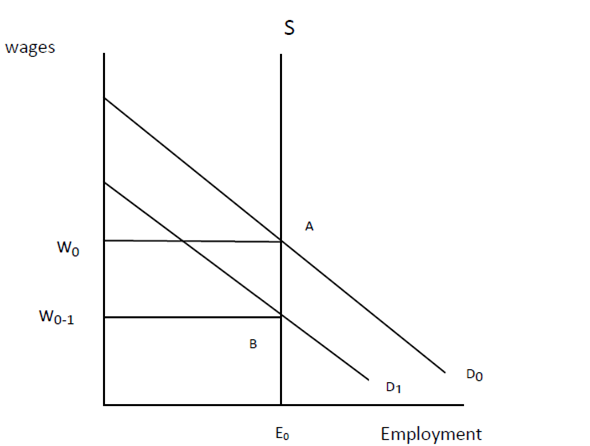# Quiz 4: Labor Market Equilibrium

Business

Let's describe the impact of the government mandates when the cost of the benefit is less than the worker's valuation.In the figure above, initially labour market is in equilibrium is point Q where both the demand curve (D 0 ) and supply curve (S 0 ) intersect each other. At this equilibrium level, the employment level is E 0 , and wage rate is w 0. Suppose the government mandates the firm to provide a particular benefit to the employees. And this benefit costs C dollars per worker to the firm, but it causes benefits B per worker, so that benefits occurring to the workers is more than the cost of the firm (B C). As a result of the increase in cost of the firm demand for labour falls, and thus the demand curve shifts downward from D 0 to D 1. Similarly, in response to the higher benefits the supply of labour increases and the supply curve shifts from S 0 to S 1. As it is clear from the figure above, the shift in supply curve is more than the shift in demand curve. Both the new demand curve and supply curve cut each other at point P which is the new equilibrium point. At this point the new employment level is E 1 , and the new wage rate is W 1. The employment level has increased, but the wage rate has decreased. However, the fall in wage rate is more than C, but less than B. Therefore, the mandated benefit is proved beneficial for both the firm and workers. There is no deadweight loss associated with the mandated benefit, because there is no fall in employment. In contrast to it, the employment has actually increased which has caused additional surplus to the firm.

Let's define Producer's surplus and Worker surplus with the help of the following figure:In the above figure, employment is shown on x axis, and wages on y axis. DD is demand curve for labour, and SS is supply curve of labour. In a competitive market, wage rate is determined by the market forces at a point where demand and supply are equal to each other. As shown in the figure, wage (w), is determined at the point where demand curve and supply curve intersect at each other. At this wage (w), the equilibrium employment level in the market is E. Now, the whole area under the demand curve represents the total revenue that has occurred to the firm. The total revenue is calculated by adding up the value of marginal product of all the workers. The value of marginal product of a labour is calculated by multiplying the price of output with marginal product of labour. Therefore, the whole area (ABC) under the demand curve is the value of marginal product of labour or total revenue of the producer. Now, the producer is paying w wages to all the workers, which is the equal to the area B and C. The remaining area (A) shows the revenue which was generated by the labour, but it remains with the producer, and this area is called the producer surplus. Along with producer surplus, workers surplus also gain in the labour market. In the above figure, the supply curve of the labour depicts the wage that is required to attract more workers in the labour market. The area C in the figure shows the workers time which could have used in alternative area, instead of supplying labour in the labour market. Now, the total area of B and C, shows the earnings of labour in the market. Therefore, triangle B shows the worker surplus, which shows how much more workers are earning than what they would have earned in other alternative uses. Thus, both producer surplus and worker surplus shows the total gains from trade that occur in a national economy. Let's show with the help of above figure that competitive market equilibrium maximizes the gains from trade: In the above figure, the labour market is in equilibrium at the point where both demand and supply curves of labour intersect each other. At this point the optimum wage is w 0 , and the equilibrium employment is E 0. It is only at this point that the gains from trade are maximum. Let's explain it why so. Suppose the employment level in the market increases from E 0 to E 2 as a result of more demand by the firms. At this point, the supply curve is above the demand curve, which shows that the value of the marginal product of the labour is less than the value of their time in some alternative use. Which means the workers would contribute more if they are put in other alternative use, therefore, the workers are not employed efficiently. In the same way, if the labour employment falls from E 0 to E 1, the gains from trade will be less than the maximum. At this point the value of marginal product of the unemployed workers is more than the value of their time in some alternative use. It means the workers would have proved more efficient if they had worked. Therefore, a competitive labour market would maximize gains from trade only when the market is in equilibrium, where demand for labour is equal to supply of labour.

In the U.S, the labour supply tends to be inelastic and the labour demand tends to be elastic, and the payroll taxes are assessed evenly between workers and firms, but in such type of situation (where the labour supply is inelastic and labour demand is elastic) the additional burden of increased payroll tax is not born evenly by the workers and firms, in fact the complete burden of payroll tax is shifted to workers only. Let's explain it with the help of following figure:As shown in the above figure, initially the elastic labour demand cuts the inelastic labour supply curve at point A where the equilibrium employment is E 0 and wage rate is w 0. Suppose government imposes a payroll tax which is expected to be shared equally by the firm and workers, but due to the inelastic supply of labour, there occur no changes in the labour supply in response to taxes. On the other hand demand for labour fall in response to payroll tax, and thus demand curve shifts inwards as D 1 curve. New lower demand curve cuts the supply curve at a lower point B. At this point the wages fall from w 0 to w 1, employment level remains same at E 0. Therefore, the additional burden of payroll tax is shifted completely to workers. If the payroll tax is assessed on just firms rather than having firms and workers sharing the burden of payroll taxes equally, even then the firms will shift the complete burden on workers given the inelastic labour supply curve. Let's explain it with the help of following figure:Initially the equilibrium is at A where the equilibrium employment level is E 0 , and equilibrium wage is w 0. Suppose a payroll tax of \$1 is imposed by the government which is to be paid by firms only. Now in the face of inelastic labour supply, the firm will reduce the demand for labour in response to the imposition of payroll tax. Therefore, the demand curve shifts inwards, and the new demand curve is D 1 , which is down by \$1. The firm is willing to pay just w 0 wages to employ E 0 workers. So, the wages are reduced to w 0 -1 , and the new equilibrium is at point B. Therefore, the burden of payroll tax is shifted completely to workers in the form of lower wages even if the government imposed the taxes on firms only.